﻿ 抗滑桩或桩板墙桩底支承错误选用的设计风险

# 抗滑桩或桩板墙桩底支承错误选用的设计风险Design Risk of Incorrect Selection of Bottom Support of Anti-Slide Pile or Pile Wall

Abstract: In the design and calculation of anti-slide piles and pile walls, many codes believe that when the rock is embedded in deep, free joints or hinge joints can be used, and some codes even support fixing. In this paper, the calculation of anti-slide piles is simulated by Midas-Civil software geotechnical calculation. The differences in the calculation results of free pile bottom, hinged pile bottom and fixed pile bottom are discussed, and the risk of wrong choice of support is put forward. It is recommended that the free end should be used in the calculation of the pile bottom constraint in the code, especially in the deformation control, the constraint of the hinged and fixed pile bottom should be deleted to avoid use the wrong model, and the actual engineering deformation is too large.

1. 引言

2. 通用有限元节点单元划分

1) 岩质地基弹簧刚度系数模拟

2) 有限元节点的岩土体横向压应力计算：

${\sigma }_{xi}={F}_{xi}/\left(n{B}_{P}\right),\text{\hspace{0.17em}}其中i=2,\cdots ,i-1$ (式-1)

${\sigma }_{si}={F}_{xi}/\left(2n{B}_{P}\right),\text{\hspace{0.17em}}其中i=1,i$ (式-2)

3. 项目概况

${K}_{s1}={K}_{si}=\text{6}0000×\text{2}.\text{5}/\left(\text{2}×\text{4}\right)=\text{1875}0\text{\hspace{0.17em}}\text{kN}/\text{m}.$

${K}_{s2}={K}_{si-1}=\text{6}0000×\text{2}.\text{5}/4=\text{375}00\text{\hspace{0.17em}}\text{kN}/\text{m}.$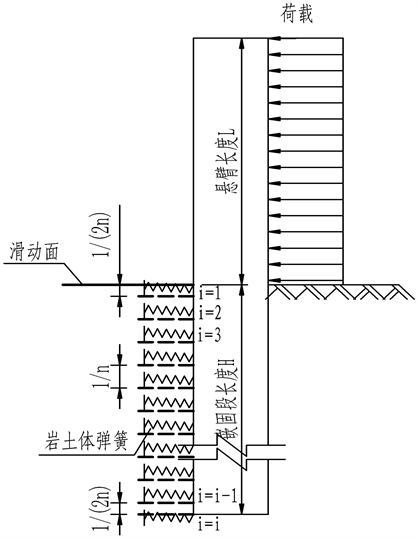Figure 1. Element division model of fixed block joint

4. Midas软件对于不同桩底约束计算结果对比分析

1) Midas计算结果

Midas软件桩底不同约束条件的弯矩汇总见图2

Midas软件桩底不同约束条件的剪力汇总见图3

Midas软件桩底不同约束条件的位移汇总见图4，反力见图5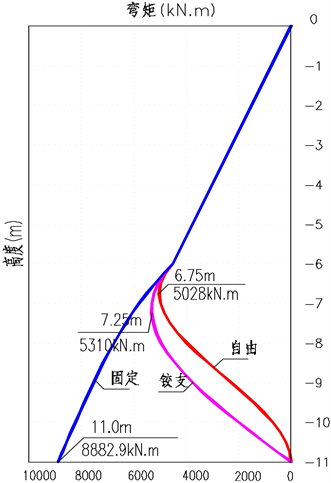Figure 2. Bending moment diagram (kN.m)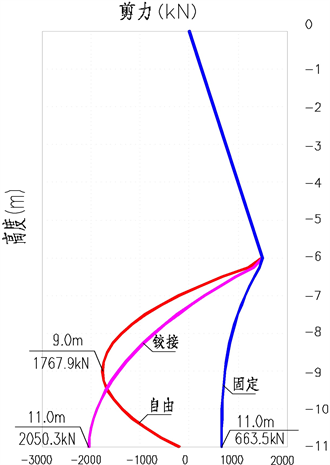Figure 3. Shear diagram (kN)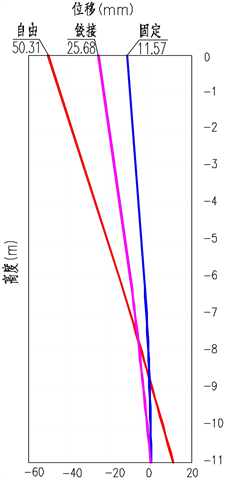Figure 4. Displacement diagram (mm)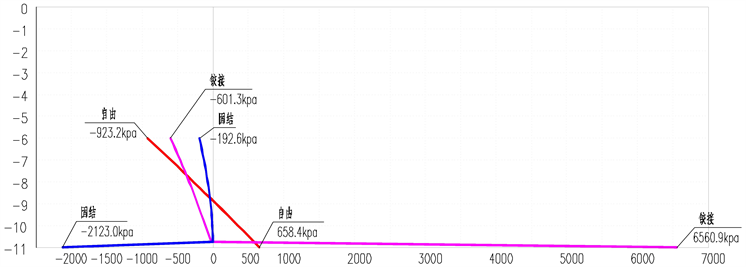Figure 5. Soil inverse force diagram (kpa)

2) 不同约束条件结果对比

5. 结论

 中华人民共和国建筑行业标准. GB/T 38509-2020滑坡防治设计规范[S]. 北京: 中国标准出版社, 2020.

 中华人民共和国建筑行业标准. DZT0219-2006滑坡防治工程设计与施工技术规范[S]. 北京: 中国标准出版社, 2006.

 黄小鹏, 杨树宝, 刘光华, 郭映忠. 嵌岩抗滑桩端支撑条件有限元分析[J]. 重庆交通大学学报(自然科学版), 2011, 30(增刊1): 526-529.

 黄钏鑫, 唐家富, 王升堂. 通用有限元模拟抗滑桩或桩板墙计算[J]. 土木工程, 2020, 9(4): 321-329.
https://doi.org/10.12677/HJCE.2020.94035

 中华人民共和国交通行业标准. JTG D30-2015公路路基设计规范[S]. 北京: 人民交通出版社股份有限公司, 2015.

Top